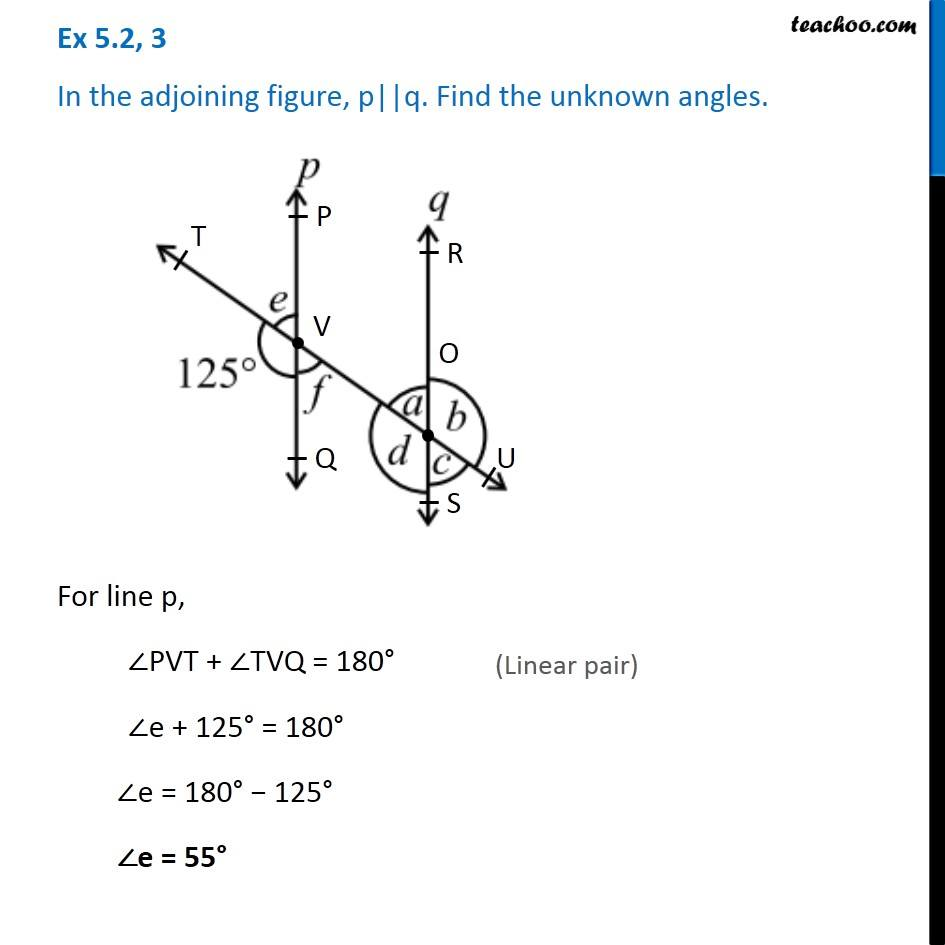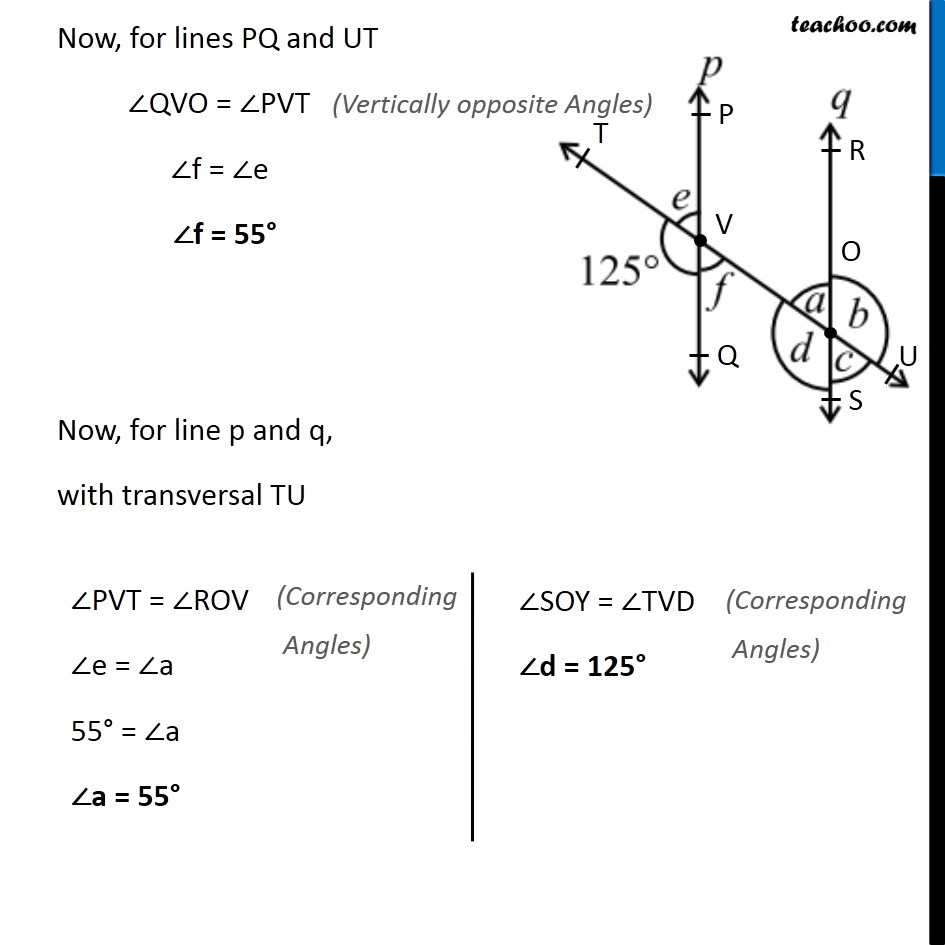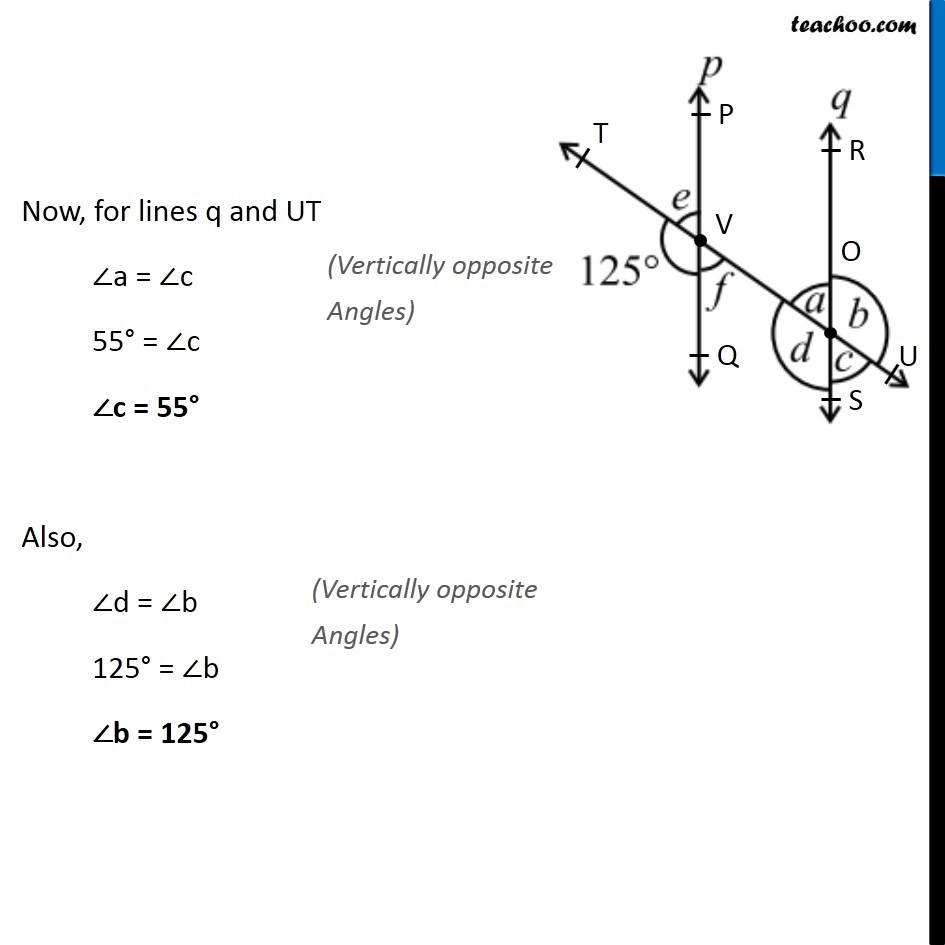Ex 5.2

Chapter 5 Class 7 Lines and Angles
Serial order wiseLearn in your speed, with individual attention - Teachoo Maths 1-on-1 Class

### Transcript

Ex 5.2, 3 In the adjoining figure, p||q. Find the unknown angles. For line p, ∠PVT + ∠TVQ = 180° ∠e + 125° = 180° ∠e = 180° − 125° ∠e = 55° Now, for lines PQ and UT ∠QVO = ∠PVT ∠f = ∠e ∠f = 55° Now, for line p and q, with transversal TU ∠PVT = ∠ROV ∠e = ∠a 55° = ∠a ∠a = 55° ∠SOY = ∠TVD ∠d = 125° Now, for lines q and UT ∠a = ∠c 55° = ∠c ∠c = 55° Also, ∠d = ∠b 125° = ∠b ∠b = 125°